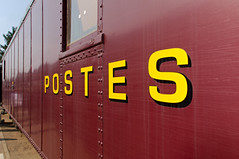Home » Ask Physics » Numerical Problems posted from Mechanics

# Numerical Problems posted from Mechanics

1. AN 8000 KG ENGINE PULLS A TRAIN OF 5 WAGONS,each of 2000 kg, along a horizontal track.if the engine exerts a force of 4000 N and the track offers a frictional force of 5000 n , then calculate
a. the net accelerating force
b.the acceleration of train
c.the force of wagon 1 on wagon 2(Posted by Fatima)

2. A truck starts from rest and rolls down a hill with a constant acceleration. It travels a distance of 400 m in 20 second .find the force acting on it if its mass is 7 metric tones (posted by Muneem)

3. Derive an equation for the constant net force acting on an object in terms of the
object’s mass, its initial velocity, its final velocity, and the time interval during which
the net force is applied. (Collin Taylor Posted)This site uses Akismet to reduce spam. Learn how your comment data is processed.

### Hits so far @ AskPhysics

• 2,274,296 hits